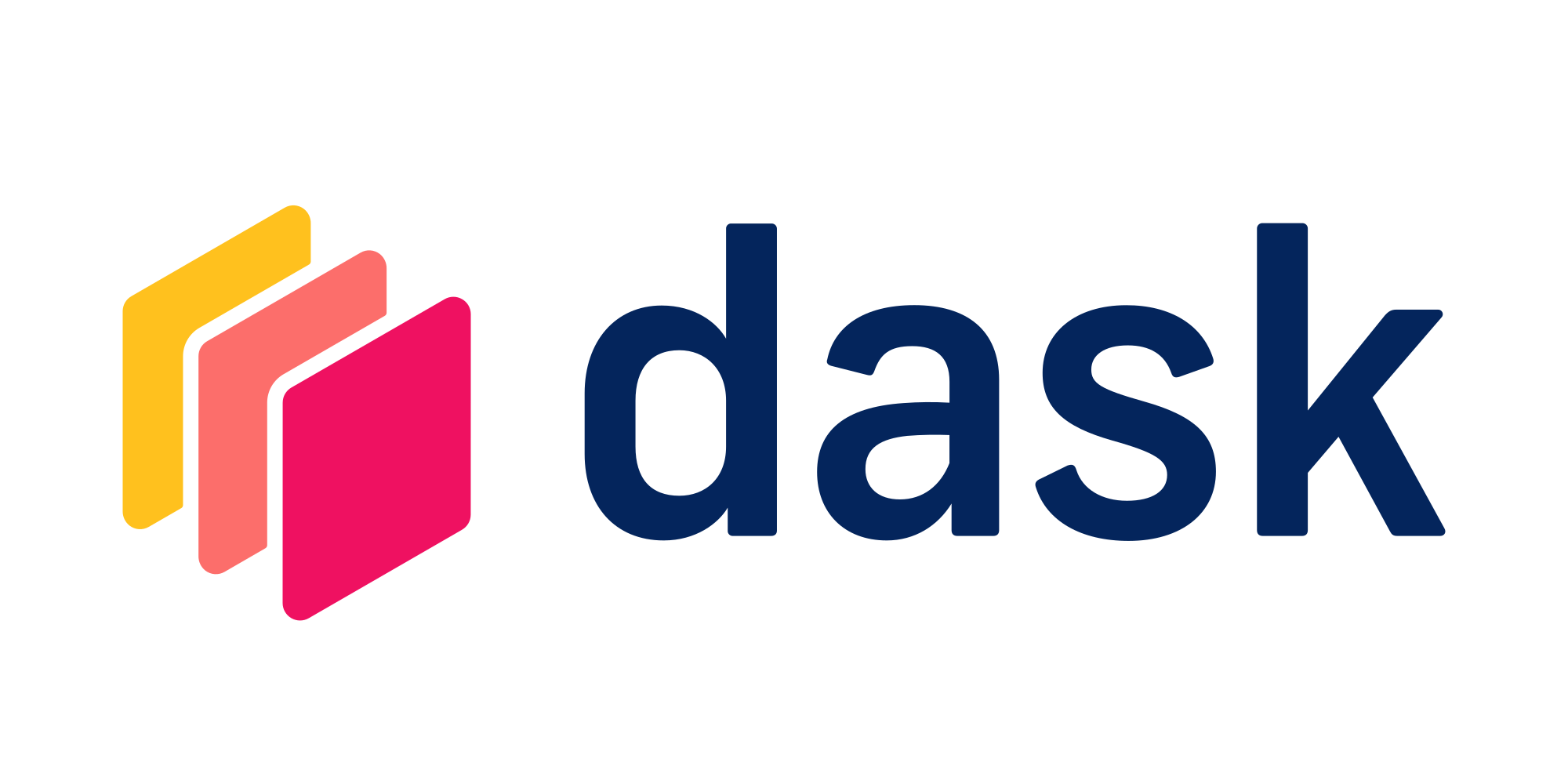Use Dask DataFrames as an alternative to pandas across multiple machinesTry this example in seconds on Saturn Cloud

If you come across problems where you find that the data is too large to fit into a pandas DataFrame or situations where computations in pandas are slow, you can transition to Dask DataFrames. Dask DataFrames mimic pandas DataFrames but allow you to distribute data across a Dask cluster.

A single Dask DataFrame can be thought of as multiple pandas DataFrames spread over multiple Dask workers. In the diagram below you can see that we have one Dask DataFrame made up of 3 pandas DataFrames, which resides across multiple machines. A pandas DataFrame can only exist on a single machine, so if the size of the data is more than the size of your machine you may come across an ‘out of memory’ error. Dask DataFrames on the other hand distributes the data, hence you can use much more data and run commands on it concurrently.

Dask DataFrames have a similar set of commands to pandas DataFrames, so creating and using them are fairly similar.

``````from dask_saturn import SaturnCluster

client = Client(SaturnCluster())
``````

After running the above command, it’s recommended that you check on the Saturn Cloud resource page that the Dask cluster as fully online before continuing. Alternatively, you can use the command `client.wait_for_workers(3)` to halt the notebook execution until all three of the workers are ready.

## Create Dask DataFrame from File

In code below, the data file is hosted on a public S3 bucket, so we can read the CSVs directly from there. Using `read_csv` from Dask takes the same form as using that function from pandas. You can also read other file formats like Parquet file, HDF files, JSON files etc. Note that Dask loads the data lazily–it won’t read in the full dataset until it is used by later operations.

``````import dask.dataframe as dd

)
``````

## Create Dask DataFrame from a pandas DataFrame

You can create a Dask DataFrame from an existing pandas DataFrame using `from_pandas`. In the code below `npartitions` states how many partitions of the index we want to create. You can also use `chunksize` parameter instead which tells the number of rows per index partition to use.

``````import pandas as pd

data = [{"x": 1, "y": 2, "z": 3}, {"x": 4, "y": 5, "z": 6}]

# Creates DataFrame.
df = pd.DataFrame(data)
df1 = dd.from_pandas(df, npartitions=1)
df1.compute()
``````

You can convert a dask array to dask dataframe using `from_dask_array` method. In code below parameter `column` lists column names for DataFrame.

``````import dask.array as da

df = dd.from_dask_array(da.zeros((9, 3), chunks=(3, 3)), columns=["x", "y", "z"])
df.compute()
``````

## Example of using Dask DataFrames

The code below shows how to do group and summary operations with Dask DataFrames. Here we have a formula one laptime dataset taken from kaggle. You can see in code below that we have used the `groupby` and `mean` functions on a Dask DataFrame the same way as we do with pandas except in the end we have added `compute()`. The `compute()` is necessary because Dask is lazy and won’t compute the operation until told to do so. When you use `read_csv` Dask DataFrame it is only going to read the column names and data types and not the entire data set. Only when you do call compute function will Dask read all the data and perform computation.

``````f1 = dd.read_csv(
)
f1.groupby("driverId").milliseconds.mean().compute()
``````

## Best Practices

1. Be thoughtful about when you use the `compute` command–a powerful part of Dask is that it can be used to avoid unnecessary computations until they’re needed.
2. Go simple whenever possible. Use Dask dataset when your data is large, but once you have filtered your data and reached a point where the data can be handled by pandas, use pandas.
3. Choose your partitions wisely. Your data in partition should be small enough to fit in memory but big enough to avoid large overheads during operations

For more details on using Dask DataFrames, see the official Dask DataFrame Documentation.

See the Saturn Cloud blog on differences in Dask and pandas for more detailed tips on this subject.

``````from dask_saturn import SaturnCluster

client = Client(SaturnCluster())

)

import pandas as pd

data = [{"x": 1, "y": 2, "z": 3}, {"x": 4, "y": 5, "z": 6}]

# Creates DataFrame.
df = pd.DataFrame(data)
df1 = dd.from_pandas(df, npartitions=1)
df1.compute()